### Home > CC3 > Chapter 7 > Lesson 7.2.3 > Problem7-66

7-66.
1. Solve each of the following equations. Be sure to show your work and check your answers. Homework Help ✎

1. 2(3x − 4) = 22

2. 6(2x − 5) = −(x + 4)

3. 2 − (y + 2) = 3y

4. 3 + 4(x + 1) = 159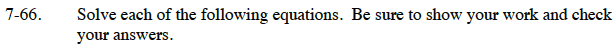Distribute, then simplify.
6x − 8 = 22
6x = 30

x = 5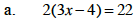Begin this problem by distributing the 6 and the negative that are outside of the parentheses, then simplify by isolating the variable onto one side of the equation.

x = 2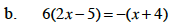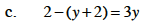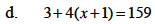Use the same methods outlined in parts (a) and (b).